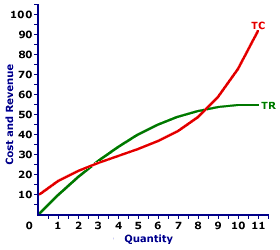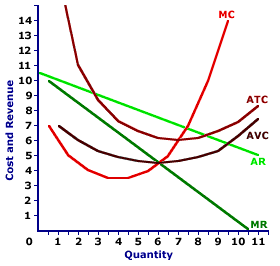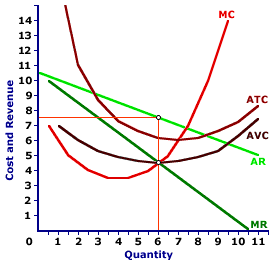Tuesday  May 30, 2023
 AmosWEB means Economics with a Touch of Whimsy!NATURAL UNEMPLOYMENT RATE: The rate of unemployment that occurs when the economy is at full employment. This rate is primarily composed of frictional and structural unemployment.MONOPOLY, SHORT-RUN PRODUCTION ANALYSIS:

A monopoly produces the profit-maximizing quantity of output that equates marginal revenue and marginal cost. This production level can be identified using total revenue and cost, marginal revenue and cost, or profit. Because a monopoly faces a negatively-sloped demand curve, it does not efficiently allocate resources by equating price and marginal cost.
Monopoly is a market structure characterized by a single seller of a unique product that has no close substitutes. These conditions mean a monopoly has complete control of the supply side of the market, which also means that the negatively-sloped market demand curve is the demand curve facing the monopoly. With this demand curve, marginal revenue is less than both average revenue and price.

Comparable to any profit-maximizing firm, a monopoly produces the quantity of output in the short run that equates marginal revenue with marginal cost. At this production level, the firm cannot increase profit by changing the level of production. Because price is not equal to marginal revenue, a monopoly does not produce the quantity of output that equates price and marginal cost, which means it is not efficiently allocating resources.

### Determining Output

A monopoly is presumed to produce the quantity of output that maximizes economic profit--the difference between total revenue and total cost. This production decision can be analyzed in three different, but interrelated ways.
• Profit: The first is directly through the analysis of economic profit, especially using a profit curve that traces the level of economic profit for different levels of output.
• Total Revenue and Cost: A second is by comparing total revenue and total cost, commonly accomplished with total revenue and total cost curves.
• Marginal Revenue and Cost: The third, and perhaps most noted, way is by comparing marginal revenue and marginal cost, similarly achieved with marginal revenue and marginal cost curves.
The profit-maximization production decision facing a monopoly can be analyzed using the exhibit below. This table presents revenue and cost information for Feet-First Pharmaceutical, a hypothetical example of a monopoly by virtue of its exclusive control over the supply of Amblathan-Plus, the only cure for the deadly (but hypothetical) foot ailment known as amblathanitis.
Profit Maximization,
Monopoly Style• Quantity: The first column presents the quantity of output produced, ranging from 0 to 12 ounces of Amblathan-Plus.

• Price: The second column presents the price received by Feet-First Pharmaceutical for selling Amblathan-Plus. As a price maker, the first and second columns represent the market demand for Amblathan-Plus. The price Feet-First Pharmaceutical faces ranges from a high of \$10.50 per ounce for a zero quantity to a low of \$4.50 per ounce for 12 ounces.

• Total Revenue: The third column presents total revenue, ranging from a low of \$0 for no output to a high of \$55 for 10 and 11 ounces, before declining for 12 ounces.

• Marginal Revenue: The fourth column presents marginal revenue, the change in total revenue due to a change in the quantity, which declines from a high of \$10 to a low of -\$1.

• Total Cost: The fifth column presents the total cost incurred by Feet-First Pharmaceutical in the production of this Amblathan-Plus, ranging from a low of \$10 for zero output (which is also fixed cost) to a high of \$117 for 12 ounces of Amblathan-Plus.

• Marginal Cost: The sixth column presents the marginal cost, the change in total cost due to a change in the quantity, which declines from \$7, reaches a low of \$3.50, then rises until reaching \$25.

• Profit: The seventh column at the far right of the table is profit, the difference between total revenue in the second column and total cost in the third column. It begins at -\$11, rises to \$8, then falls to -\$63.
The choice facing Feet-First Pharmaceutical is to produce the quantity of Amblathan-Plus that maximizes profit. This can be easily identified using the far right "Profit" column. The quantity that generates the greatest level of economic profit is 6 ounces of Amblathan-Plus. This alternative can be highlighted by clicking the [Profit Max] button.

The production of 6 ounces of Amblathan-Plus results in \$45 of total revenue and \$37 of total cost, a difference of \$8. No other production level generates a greater economic profit. Producing one more ounce of Amblathan-Plus or one less ounce of Amblathan-Plus reduces profit to \$7. The price that achieves this profit-maximizing quantity is \$7.50.

While marginal revenue and marginal cost might not appear to be equal for the profit-maximizing 6 ounces of Amblathan-Plus production (\$5 versus \$4), they really are. The reason is that the marginal numbers in the table actually represent discrete changes from one ounce to the next. Reducing the size of the discrete change, say from 5.9999 ounces to 6 ounces, results in a marginal cost that is closer to \$4.50. The same is true for marginal cost. At the limit of an infinitesimally small change, both marginal cost and marginal revenue are exactly \$4.50.

### Total Curves

Total Revenue and CostThe short-run production decision for a monopoly can be graphically illustrated using total revenue and total cost curves, such as those displayed in the exhibit to the right. These total curves represent the total revenue and cost of Amblathan-Plus production by Feet-First Pharmaceutical the Amblathan-Plus grower.
• Total Revenue: The hump-shaped green line (TR) depicts the total revenue Feet-First Pharmaceutical receives from Amblathan-Plus production. The line is hump-shaped because Feet-First Pharmaceutical must lower the price to sell a larger quantity.

• Total Cost: The curvy red line (TC) depicts the total cost Feet-First Pharmaceutical incurs in the production of Amblathan-Plus. The shape is based on increasing, then decreasing marginal returns.

• Profit: The vertical difference between these two lines is economic profit. If the total revenue line is above the total cost line in the middle of the diagram, economic profit is positive. If the total revenue line is below the total cost line at the far right and far left, economic profit is negative.
The key for Feet-First Pharmaceutical is to identify the production level that gives the greatest vertical distance between the total revenue and total cost curves in the middle of the diagram. This might not be evident by just looking at the exhibit, but it can be illustrated by clicking the [Profit Max] button. The output quantity identified is, once again, 6 ounces of Amblathan-Plus.

### Marginal Curves

Marginal Revenue and CostPerhaps the most common method of identifying the profit-maximizing level of production for a monopoly is using marginal revenue and marginal cost curves, such as those displayed in this exhibit.
• Marginal Revenue: The negatively-sloped dark green line, labeled MR, is the marginal revenue Feet-First Pharmaceutical receives for each extra ounce of Amblathan-Plus sold.

• Marginal Cost: The red U-shaped curve, labeled MC, is the marginal cost Feet-First Pharmaceutical incurs in the production of Amblathan-Plus. The shape is based on increasing, then decreasing marginal returns.

• Average Revenue: The negatively-sloped light green line (AR) is the average revenue Feet-First Pharmaceutical receives from selling Amblathan-Plus, which is also the demand curve.

• Average Cost: Two additional U-shaped curves included in the diagram (just for good measure) are average total cost (ATC) and average variable cost (AVC). These curves are helpful when identifying the level of economic profit or loss and the firm's short-run supply curve.
In this analysis, Feet-First Pharmaceutical needs to identify the quantity of output that achieves an equality between marginal revenue and marginal cost. This production can be highlighted by clicking the [Profit Max] button. The highlighted quantity is once again 6 ounces of Amblathan-Plus. The price charged by Feet-First Pharmaceutical to sell this quantity is \$7.50.

### Production Alternatives

Profit and LossEven though monopoly is more likely to earn economic profit than a perfectly competitive firm, it is not guaranteed an economic profit. Should demand conditions change, monopoly might incur an economic loss or be forced to shut down in the short run. Comparable to any firm, a monopoly faces three short-run production alternatives based on a comparison of price, average total cost, and average variable cost.
• P > ATC: Total revenue exceeds total cost and Feet-First Pharmaceutical receives a positive economic profit. In this case, Feet-First Pharmaceutical maximizes profit by producing the quantity of output that equates marginal revenue and marginal cost. More to the point, the price that Feet-First Pharmaceutical charges at the profit-maximizing quantity is greater than average total cost. This is the initial situation displayed in the graph.

• ATC > P > AVC: Total revenue falls short of total cost, meaning Feet-First Pharmaceutical incurs an economic loss (or negative economic profit). This option can be displayed by clicking the [Less Demand] button. In spite of the loss, because the price set at the profit-maximizing production level exceeds average variable cost, Feet-First Pharmaceutical can minimize loss by producing the indicated quantity of output. Feet-First Pharmaceutical receives enough revenue to cover ALL variable cost plus a portion of fixed cost. The loss from production is less than the loss from NOT producing, which is total fixed cost.

• P < AVC: Total revenue also falls short of total cost, and Feet-First Pharmaceutical incurs an economic loss (or negative economic profit) that exceeds total fixed cost. This option can be displayed by clicking the [Even Less Demand] button. In this case Feet-First Pharmaceutical minimizes losses by reducing the quantity of output to zero, or producing no output in the short run. By producing a positive quantity, Feet-First Pharmaceutical does not receive enough revenue to cover variable cost, let alone any fixed cost. The economic loss from producing is greater than the economic loss of NOT producing, which is total fixed cost.

### Short-Run Supply Curve?

Market control means that monopoly does necessarily not have a supply relation between the quantity of output produced and the price. By way of comparison a perfectly competitive firm has a short-run supply curve based on the positively sloped marginal cost curve. A perfectly competitive firm's supply curve is that portion of the marginal cost curve that lies above the minimum of the average variable cost curve. A perfectly competitive firm maximizes profit by producing the quantity of output that equates price and marginal cost. As such, the firm moves along the marginal cost curve in response to alternative prices.

However, market control means that price is NOT equal to marginal revenue, and thus monopoly does NOT equate marginal cost and price. As such, a monopoly firm does not move along the marginal cost curve. A monopoly does not necessarily supply larger quantities at higher prices or smaller quantities at lower prices.

As a price maker that controls the market, a monopoly reacts to demand conditions, especially the price elasticity of demand, when setting the price and corresponding quantity produced. While it is not out of the question for a monopoly to supply a larger quantity at a higher price, it is also conceivable that a monopoly produces a smaller quantity at a higher price or a larger quantity at a lower price.

The bottom line is that monopoly does not necessarily have a short-run supply curve relation.

### (In)Efficiency

(In)EfficiencyBecause a monopoly charges a price that exceeds marginal cost, it does efficiently allocates resources. Price represents the value of goods produced and marginal cost represents the value of goods not produced. When both are equal, satisfaction cannot be increased by changing production.

However, because price exceeds marginal cost, the economy gives up less satisfaction from other goods not produced than it receives from the good that is produced. The economy can gain satisfaction by producing more of the good.

This exhibit once again displays the profit-maximizing production output of Feet-First Pharmaceutical. Feet-First Pharmaceutical produces the quantity (6 ounces) of Amblathan-Plus that equates marginal revenue and marginal cost. The resulting price charge is \$7.50 per ounce.

This price exceeds marginal cost, meaning Feet-First Pharmaceutical is inefficient. The price is higher and the quantity produced is less than what would be achieved with an efficient production.

To see why, consider how an efficient allocation would look. This alternative can be displayed with a click of the [Efficient] button. This efficient production level occurs at the intersection of the marginal cost curve (MC) and the demand/average revenue curve (AR). The efficient price is just under \$7 per ounce and the efficient quantity produced is almost 7.5 ounces. At this production level, price is equal to marginal cost.

In comparison, the price charged by the monopoly is higher and the quantity produced is less.

 <= MONOPOLY, REVENUE DIVISION MONOPOLY, SHORT-RUN SUPPLY CURVE =>Recommended Citation:

MONOPOLY, SHORT-RUN PRODUCTION ANALYSIS, AmosWEB Encyclonomic WEB*pedia, http://www.AmosWEB.com, AmosWEB LLC, 2000-2023. [Accessed: May 30, 2023].

Check Out These Related Terms...

Or For A Little Background...

And For Further Study...
Search Again?BLACK DISMALAPOD[What's This?] Today, you are likely to spend a great deal of time browsing through a long list of dot com websites trying to buy either arch supports for your shoes or an AC adapter that works with your MPG player. Be on the lookout for strangers with large satchels of used undergarments.Your Complete ScopeNorth Carolina supplied all the domestic gold coined for currency by the U.S. Mint in Philadelphia until 1828."Never let the fear of striking out get in your way. "-- Babe RuthDJDow JonesA PEDestrian's Guide Xtra CreditTell us what you think about AmosWEB. Like what you see? Have suggestions for improvements? Let us know. Click the User Feedback link.| | | | | | | | | | |
| | | |

Thanks for visiting AmosWEB